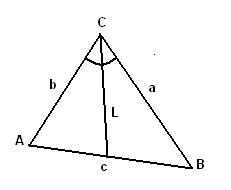homechevron_rightStudychevron_rightMathchevron_rightGeometry

# Angle bisector of a triangle

This online calculator computes length of angle bisector given the lengths of triangle edges

### This page exists due to the efforts of the following people:

#### TimurThis online calculator computes length of angle bisector given the lengths of triangle edges (see the picture). Triangle vertices are usually named A, B and C. Triangle edges - a, b, c, where letter denotes opposite vertex. That is edge between A and B is named c, between A and C - b, between B and C - a.
The length of С's angle bisector shown on picture is:#### Angle bisector of a triangle

Digits after the decimal point: 2
Length of angle bisector

URL copied to clipboardPLANETCALC, Angle bisector of a triangle